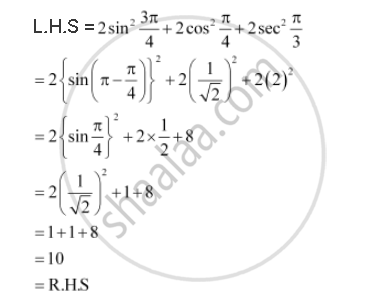CBSE (Arts) Class 11CBSE
Share

# Prove That 2 Sinsqrt2 (3pi)/4 + 2 Cossqrt2 Pi/4 + 2 Secsqrt2 Pi/3 = 10 - CBSE (Arts) Class 11 - Mathematics

ConceptTrigonometric Functions of Sum and Difference of Two Angles

#### Question

Prove that  2 sin^2  (3pi)/4 + 2 cos^2  pi/4  + 2 sec^2  pi/3  = 10

#### SolutionIs there an error in this question or solution?

#### APPEARS IN

NCERT Solution for Mathematics Textbook for Class 11 (2018 to Current)
Chapter 3: Trigonometric Functions
Q: 4 | Page no. 73

#### Video TutorialsVIEW ALL 

Solution Prove That 2 Sinsqrt2 (3pi)/4 + 2 Cossqrt2 Pi/4 + 2 Secsqrt2 Pi/3 = 10 Concept: Trigonometric Functions of Sum and Difference of Two Angles.
S